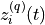# control.flatsys.BasisFamily¶

class control.flatsys.BasisFamily(N)[source]

Bases: `object`

Base class for implementing basis functions for flat systems.

A BasisFamily object is used to construct trajectories for a flat system. The class must implement a single function that computes the jth derivative of the ith basis function at a time t:= basis.eval_deriv(self, i, j, t)

A basis set can either consist of a single variable that is used for each flat output (nvars = None) or a different variable for different flat outputs (nvars > 0).

N

Order of the basis set.

Type

int

Methods

 `eval` Compute function values given the coefficients and time points. `eval_deriv` Evaluate the kth derivative of the ith basis function at time t. `var_ncoefs` Get the number of coefficients for a variable
__call__(i, t, var=None)[source]

Evaluate the ith basis function at a point in time

eval(coeffs, tlist, var=None)[source]

Compute function values given the coefficients and time points.

eval_deriv(i, j, t, var=None)[source]

Evaluate the kth derivative of the ith basis function at time t.

var_ncoefs(var)[source]

Get the number of coefficients for a variable